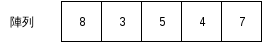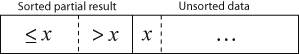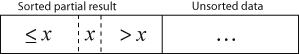#[Rust] 程式設計教學：陣列 (Array)、向量 (Vector)和切片 (Slice)

## 陣列### 建立陣列

``````fn main() {
let array = [1, 2, 3, 4, 5];
}``````

``````fn main() {
const SIZE: usize = 5;

// [0.0, 0.0, 0.0, 0.0, 0.0]
let mut array: [f64; SIZE] = [0.0; SIZE];
}``````

C 或 C++ 可用動態配置記憶體産生陣列，在 Rust 中相對應的動作是使用向量 (vector)。向量是一種可動態改變大小的線性容器，我們將於下文介紹。

### 存取陣列資料

``````fn main() {
let array = [1, 2, 3];

assert_eq!(array, 1);
assert_eq!(array, 2);
assert_eq!(array, 3);
}````````````fn main() {
let mut array = [0; 3];

array = 1;
array = 2;
array = 3;

assert_eq!(array, 1);
assert_eq!(array, 2);
assert_eq!(array, 3);
}``````

### 走訪陣列

``````fn main() {
// Numbers in German
let array = ["eins", "zwei", "drei", "vier", "fünf"];

for i in 0..array.len() {
println!("{}", array[i]);
}
}``````

``````fn main() {
let array = ["eins", "zwei", "drei", "vier", "fünf"];

for element in array.iter() {
println!("{}", element);
}
}``````

``````fn main() {
let array = ["eins", "zwei", "drei", "vier", "fünf"];

// Error
for element in array {
println!("{}", element);
}
}``````

``error[E0277]: the trait bound `[&str; 5]: std::iter::Iterator` is not satisfied``

``````// Call rand package for random number generation
extern crate rand;

use rand::Rng;

fn main() {
const SIZE: usize = 10;
let mut array = [0; SIZE];

for i in 0..array.len() {
// Set a random number between 1 and 100
array[i] = rand::thread_rng().gen_range(1, 100 + 1);
}
}``````

``````extern crate rand;

use rand::Rng;

fn main() {
const SIZE: usize = 100;
let mut array = [0; SIZE];

for e in array.iter_mut() {
*e = rand::thread_rng().gen_range(1, 100 + 1);
}
}``````

### 陣列的限制

``````extern crate rand;

use rand::Rng;

fn main() {
const SIZE: usize = 33;  // Watch out when SIZE > 32
const MIN: i32 = 1;
const MAX: i32 = 100;

let mut array: [i32; SIZE] = [0; SIZE];

for i in 0..SIZE {
array[i] = rand::thread_rng().gen_range(MIN, MAX + 1);
}

println!("{:?}", array);  // Error when SIZE > 32
}``````

``error[E0277]: the trait bound `[i32; 33]: std::fmt::Debug` is not satisfied``

1. 自行實作相關 trait
2. 避免使用這些方法
3. 改用向量

(1) 不是通用的方法，因為針對每個長度，都要重新實作一次，但若有需求，仍可考慮；(2) 則會限制了陣列的使用場合；通常可考慮 (3)。

## 向量

### 建立向量

``````fn main() {
let vec = vec![1, 2, 3];
}``````

``````fn main {
/* Type inference works here,
so we don't explicitly declare
the type of vec. */
let mut vec = Vec::new();  // vec<i32>

// Append data into the tail of the vector
vec.push(1);
vec.push(2);
vec.push(3);
}``````

`push` 的概念是，從向量尾端附加一個新的元素，就像是在一列火車尾端掛載一節車箱般。Rust 的向量從尾端加入資料的效率相當好，可視為常數時間。

### 存取向量資料

``````fn main() {
let vec = vec![1, 2, 3];

assert_eq!(vec, 1);
assert_eq!(vec, 2);
assert_eq!(vec, 3);
}``````

``````fn main() {
let mut vec = vec![1, 2, 3];

vec = 99;  // Feed data into vector

assert_eq!(vec, 1);
assert_eq!(vec, 99);
assert_eq!(vec, 3);
}``````

### 走訪向量

``````fn main() {
let vec = vec![1, 2, 3];

for i in 0..(vec.len()) {
println!("{}", i);
}
}``````

``````fn main() {
let vec = vec![1, 2, 3];

for element in vec.iter() {
println!("{}", element);
}
}``````

``````fn main() {
let mut vec = vec![1, 2, 3];

for i in 0..vec.len() {
vec[i] = vec[i] * vec[i];
}

assert_eq!(vec, 1);
assert_eq!(vec, 4);
assert_eq!(vec, 9);
}``````

``````fn main() {
let mut vec = vec![1, 2, 3];

for element in vec.iter_mut() {
*element = (*element) * (*element);
}

assert_eq!(vec, 1);
assert_eq!(vec, 4);
assert_eq!(vec, 9);
}``````

### 一些操作向量的方法

``````fn main() {
// Declare an empty vector
let mut vec = Vec::new();

// Append data to the tail of the vector
vec.push(1);
vec.push(2);
vec.push(3);

// Get the length of the vector
assert_eq!(vec.len(), 3);

// Pop data from the tail of the vector
let popped = vec.pop().unwrap();
assert_eq!(popped, 3);
assert_eq!(vec, vec![1, 2]);

// Insert data into the middle of the vector
vec.insert(1, 99);
assert_eq!(vec, vec![1, 99, 2]);

// Remove data from the middle of the vector
let removed = vec.remove(1);
assert_eq!(removed, 99);
}``````

`vec.pop().unwrap()` 這個部分的程式碼可能會令讀者困惑，這是 Rust 的特殊容器 Option。該容器的用途是為了處理錯誤情形，在從向量尾端取出資料時，有可能取出的值為空值，故 Rust 將值包裝在該容器中。這部分牽涉到 enum 的概念，將於後續章節中說明。

## 切片

### 建立切片

``````fn main() {
/* Internally, it works as this:
let _slice = [1, 2, 3, 4, 5];
let slice = &_slice; */
let slice = &[1, 2, 3, 4, 5];
}``````

### 存取切片資料

``````fn main() {
let slice = &[1, 2, 3];

assert_eq!(slice, 1);
assert_eq!(slice, 2);
assert_eq!(slice, 3);
}``````

``````fn main() {
let slice = &mut [1, 2, 3];

// Write data into slice
slice = 99;

assert_eq!(slice, 1);
assert_eq!(slice, 99);
assert_eq!(slice, 3);
}``````

### 走訪切片

``````fn main() {
let array = ["eins", "zwei", "drei", "vier", "fünf"];

// It works when the array size <= 32
for element in &array {
println!("{}", element);
}
}``````

``````extern crate rand;

use rand::Rng;

fn main() {
const SIZE: usize = 10;
let mut array = [0; SIZE];

for element in &mut array {
*element = rand::thread_rng().gen_range(1, 100 + 1);
}
}``````

## (案例選讀) 插入排序法``````Let A a zero-based array with size n

for i from 1 to n - 1 {
x = A[i]
j = i
while j > 0 and A[j-1] > x {
A[j] = A[j-1]
j = j - 1
}
A[j] = x
}``````

``````// Call rand library
extern crate rand;

use rand::Rng;

fn main() {
// Initialize variables
const SIZE: usize = 10;
let mut array: [i32; SIZE] = [0; SIZE];

// Set array elements with random integers
for i in 0..SIZE {
array[i] = rand::thread_rng().gen_range(1, 100 + 1);
}

// Print out unsorted array
print!("Before sort: ");
display_slice(&array);

// Insertion sort.
// Modify the array in-place.
for i in 1..(array.len()) {
let x = array[i];  // Temp data
let mut j = i;
while j > 0 &&  array[j-1] > x {
array[j] = array[j-1];  // Move element one step
j -= 1;
}
array[j] = x;  // Put back temp data
}

// Print out sorted array
print!("After sort: ");
display_slice(&array);
}

// Function to print out array with arbitrary size
fn display_slice(slice: &[i32]) {
for i in 0..slice.len() {
print!("{}", slice[i]);

if i < slice.len() - 1 {
print!(", ");
}
}
println!("");
}``````

• Bubble sort
• Selection sort
• Bucket sort